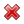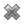# Fight Finance

#### CoursesTagsRandomAllRecentScoresScoreskeithphw $6,011.61 Jade$1,815.80 Chu $789.98 royal ne...$750.00 Leehy $713.33 Visitor$650.00 JennyLI $625.61 Visitor$590.00 ZOE HY $560.00 Visitor$555.33 Visitor $550.00 Visitor$550.00 Visitor $540.00 Visitor$500.00 Yizhou $489.18 Visitor$480.00 Visitor $464.70 Visitor$460.00 Visitor $460.00 Visitor$460.00

A European company just issued two bonds, a

• 2 year zero coupon bond at a yield of 8% pa, and a
• 3 year zero coupon bond at a yield of 10% pa.

What is the company's forward rate over the third year (from t=2 to t=3)? Give your answer as an effective annual rate, which is how the above bond yields are quoted.

A European company just issued two bonds, a

• 1 year zero coupon bond at a yield of 8% pa, and a
• 2 year zero coupon bond at a yield of 10% pa.

What is the company's forward rate over the second year (from t=1 to t=2)? Give your answer as an effective annual rate, which is how the above bond yields are quoted.

An Australian company just issued two bonds:

• A 1 year zero coupon bond at a yield of 8% pa, and
• A 2 year zero coupon bond at a yield of 10% pa.

What is the forward rate on the company's debt from years 1 to 2? Give your answer as an APR compounding every 6 months, which is how the above bond yields are quoted.

An Australian company just issued two bonds:

• A 1 year zero coupon bond at a yield of 10% pa, and
• A 2 year zero coupon bond at a yield of 8% pa.

What is the forward rate on the company's debt from years 1 to 2? Give your answer as an APR compounding every 6 months, which is how the above bond yields are quoted.

An Australian company just issued two bonds:

• A 6-month zero coupon bond at a yield of 6% pa, and
• A 12 month zero coupon bond at a yield of 7% pa.

What is the company's forward rate from 6 to 12 months? Give your answer as an APR compounding every 6 months, which is how the above bond yields are quoted.

In the below term structure of interest rates equation, all rates are effective annual yields and the numbers in subscript represent the years that the yields are measured over:

$$(1+r_{0-3})^3 = (1+r_{0-1})(1+r_{1-2})(1+r_{2-3})$$

Which of the following statements is NOT correct?

In the below term structure of interest rates equation, all rates are effective annual yields and the numbers in subscript represent the years that the yields are measured over:

$$(1+r_{0-3})^3 = (1+r_{0-1})(1+r_{1-2})(1+r_{2-3})$$

Which of the following statements is NOT correct?